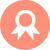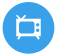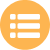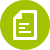## 二建-建筑工程## 监理案例分析# 陕西省西安信号工在线考核试题

A.15t

B.16～20t

C.26t

D.35t

A.安全作业范围(环境)

B.安全作业高度(高程)

C.安全运转速度(时间)

D.安全作业距离(幅度)

A.独立高度

B.悬臂高度

C.空载高度

D.荷载高度

A.预备

B.要主钩

C.要副钩

D.吊钩上升

A.产品标牌

B.产品铭牌

C.产品说明书

D.产品合格证

A.生活区应当建立消防责任制

B.生活区内应设置消火栓或不小于20m3容量的蓄水池蓄水

C.宿舍内严禁作用电炉等电加热器具

D.宿舍内严禁吸烟

A.上行行程限位

B.下行行程限位

C.平行行程限位

D.垂直行程限位

A.保养润滑

B.技术修理

C.更换或报废

D.维护保养

A.70%

B.75%

C.80%

D.85%

A.使用移动梯和折梯

B.安装外墙门窗

C.交叉作业中拆卸模板.脚手架

D.给水平面洞口设置封口盖板

A.可能松散

B.可能断股

C.可能断丝

D.可能滑移

A.安全文化

B.安全投入

C.安全法制

D.安全责任心

A.平地机

B.塔式起重机

C.挖掘机

D.装载机

A.大于

B.等于

C.小于

D.大于等于

A.强度改变

B.强度下降

C.强度突变

D.强度上升

A.主要原因

B.次要原因

C.相关原因

D.其他原因

A.顶部

B.低部

C.左边

D.右边

A.分开设置

B.合并设置

C.上下设置

D.左右设置

A.3

B.4

C.5

D.6

A.转臂

B.微微伸臂

C.升臂

D.降臂

A.-10℃

B.-5℃

C.-20℃

D.0℃

A.通道处不得堆放障碍物

B.不得在室内配制用于防腐.防水.装饰的危险化学品溶液

C.施工人员吸烟和动火应先申请

D.不得同时进行水暖.电气安装的金属焊割作业

A.起重臂下

B.作业半径

C.起重旋转半径

D.吊装区域

A.保持缓慢弯曲

B.保持不弯曲

C.向左右两个方向弯曲

D.向一个方向弯曲

A.中心

B.重心

C.形心

D.质心

A.二氧化碳灭火器约为2.5m

B.四氯化碳灭火器约为3m

C.干粉灭火器约为4.5m

D.1211灭火器约为7m

A.安全性检查

B.符合性检查

C.准备性检查

D.实质性检查

A.进行维护

B.进行确认

C.进行检修

D.进行维修

A.时松时紧

B.始终松弛

C.始终紧张

D.可松可紧

A.安全生产管理机构负责人

B.分管安全工作的副经理

C.法定代表人

D.企业技术负责人

A.在潮湿场所或金属构架上严禁使用ⅱ类手持式电动工具

B.狭窄场所必须选用由安全隔离变压器供电的ⅲ类手持式电动工具

C.外壳.手柄.插头.开关.负荷线等必须完好无损

D.负荷线应釆用耐气候的橡皮护套铜芯软电缆，并不得有接头

A.专职安全生产管理人员

B.施工员

C.项目技术负责人

D.专人

A.建设主管部门

B.所在单位

C.行业协会

D.劳动主管部门

A.10%

B.20%

C.30%

D.40%

A.不要重复

B.重复一次

C.重复2次

D.复述一次

A.柱销

B.卸扣

C.横吊梁

D.棕绳

A.30°

B.45°

C.60°

D.75°

A.蜗杆传动

B.齿条传动

C.圆柱传动

D.电动传动

A.旋转法

B.递送法

C.平移法

D.滑移法

A.局部压缩

B.局部变形

C.局部松散

D.局部破裂

A.1～1.5m

B.1.5～2m

C.2～2.5m

D.2.5～3m

A.停止起重机作业

B.停止吊装作业

C.停止所有作业

D.停止起重作

A.平头式

B.锤头式

C.折臂式

D.动臂式

A.戴防护手套

B.系好安全带

C.戴防尘口罩

D.戴防护眼镜

A.一定要有良好的通风条件

B.照明电气设备必须使用防爆灯头

C.严禁吸烟

D.周围动火作业要距离15m以外

A.伸臂

B.微微伸臂

C.微微降臂

D.微微转臂

A.通用手势

B.专用手势

C.旗语信号

D.专用信号

A.5日

B.10日

C.20日

D.30日

A.通用手势

B.专用手势

C.特殊手势

D.专用音响

A.潜在的隐患

B.危害的信号

C.报废的信号

D.风险的信号

A.采用斜拉

B.采用斜吊

C.采用直吊

D.采用平吊

E.缓慢吊运

A.长方形构件

B.弯曲形构件

C.无横向对称面构件

D.无纵向对称面构件

E.体形复杂构件

A.合成纤维吊装带

B.钢丝绳吊索

C.吊链

D.其他连接件

E.安全锁

A.固定式塔式起重机

B.附着式塔式起重机

C.行走式塔式起重机

D.内爬式塔式起重机

E.支腿式塔式起重机

A.保证可靠

B.摘钩简便

C.操作过程

D.使用过程

E.人员下班

A.禁止标志

B.警告标志

C.指令标志

D.醒目标志

E.提示标志

A.年龄及身体状况

B.毒品名称及剂量

C.中毒时间

D.曾否呕吐

E.清醒程度

A.单机吊装

B.双机抬吊

C.三机抬吊

D.相互抬吊

E.多机抬吊

A.转臂

B.微微降臂

C.起重机前进

D.吊钩微微下降

E.指示降落方位

A.同时报废

B.同时设计

C.同时施工

D.同时投入生产和使用

E.同时竣工

A.缆风绳

B.支撑

C.钢钎

D.屋架校正器

E.楔子

A.塑料

B.植物产品

C.油脂

D.煤

E.硫化铁

A.直径

B.几股几丝

C.芯材

D.钢丝强度

E.安全系数

A.口服

B.吸入

C.皮肤吸收

D.注射

E.接触

A.搁置点

B.拉结点

C.支撑点

D.锚固点

E.附加点

A.插接

B.打结

C.绳扣

D.螺栓

E.绳卡

A.手拳

B.手指

C.手臂

D.手心

E.臂部

A.防雨(电焊机特别应加防护雨罩)

B.防潮

C.防淹

D.防风

E.防雷

A.采用三级配电系统

B.采用tn-s接零保护系统

C.采用二级漏电保护系统

D.采用三相五线制

E.“一机一箱”制

A.超高层建筑

B.小型工程

C.大型工程

D.高层建筑

E.大型多机吊运

A.正确

B.错误

A.正确

B.错误

A.正确

B.错误

A.正确

B.错误

A.正确

B.错误

A.正确

B.错误

A.正确

B.错误

A.正确

B.错误

A.正确

B.错误

A.正确

B.错误

A.正确

B.错误

A.正确

B.错误

A.正确

B.错误

A.正确

B.错误

A.正确

B.错误

A.正确

B.错误

A.正确

B.错误

A.正确

B.错误

A.正确

B.错误

A.正确

B.错误

##### 版权声明

 留言与评论（共有 0 条评论）

ock123.com

### 蜀ICP备18034711号.